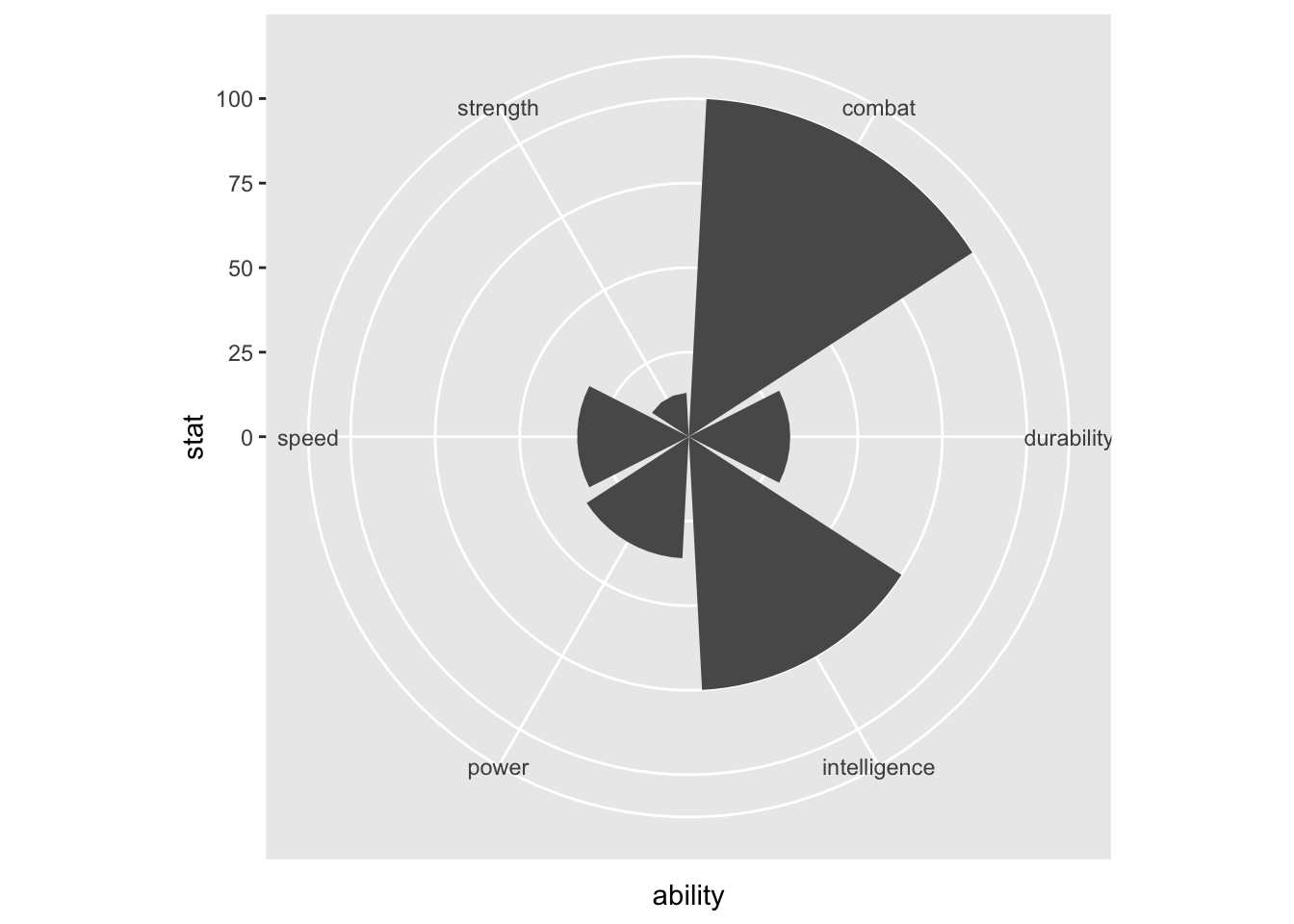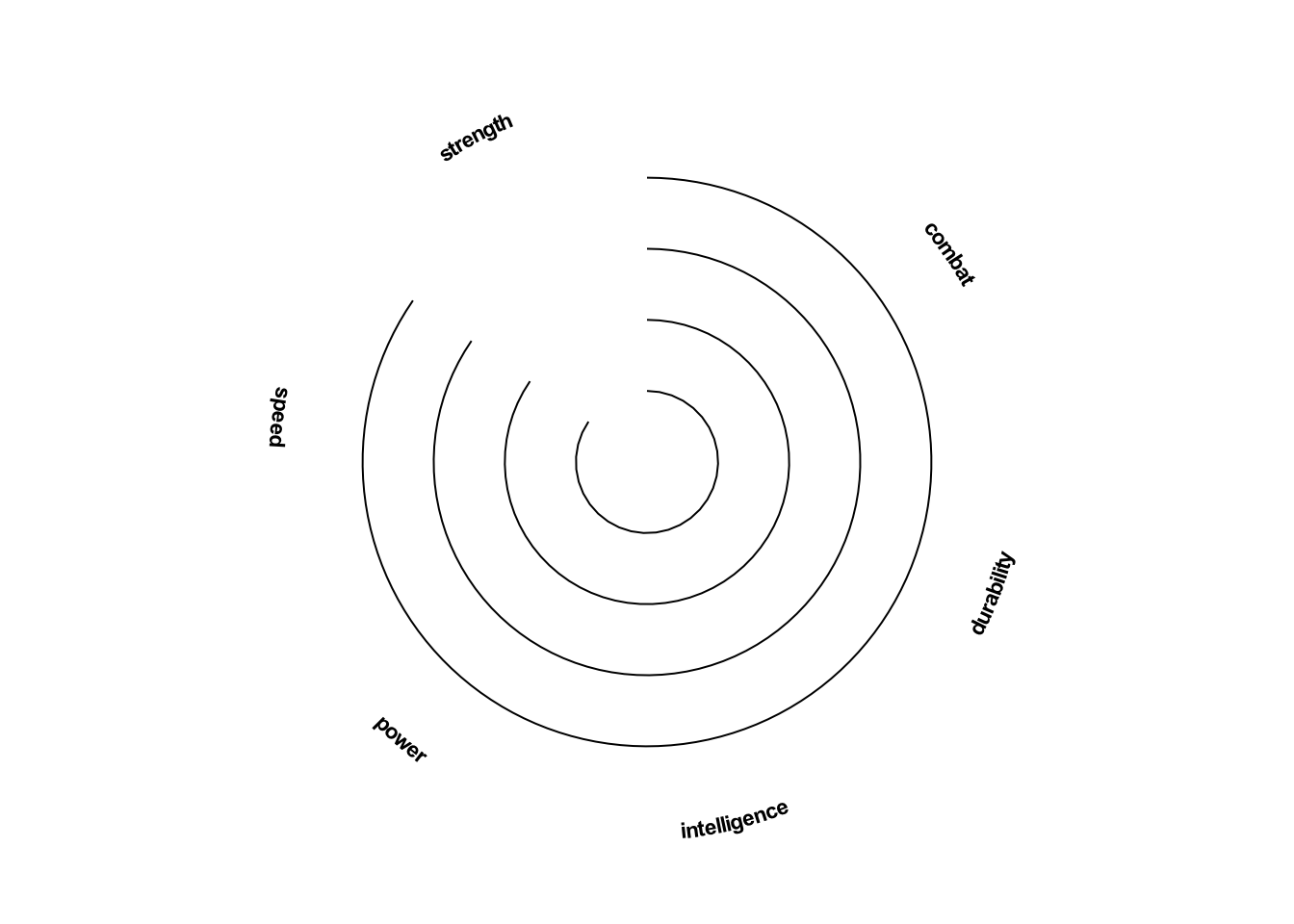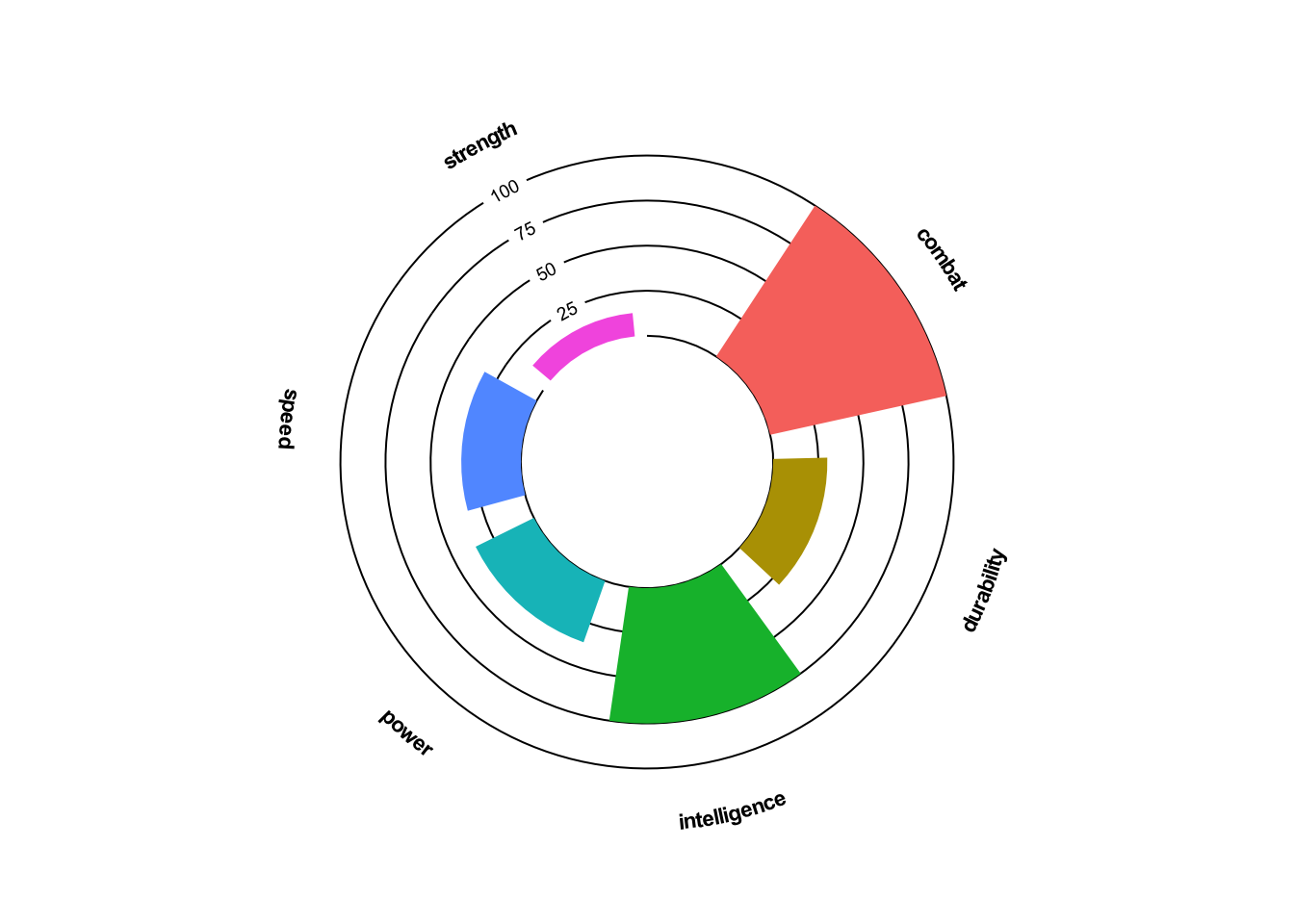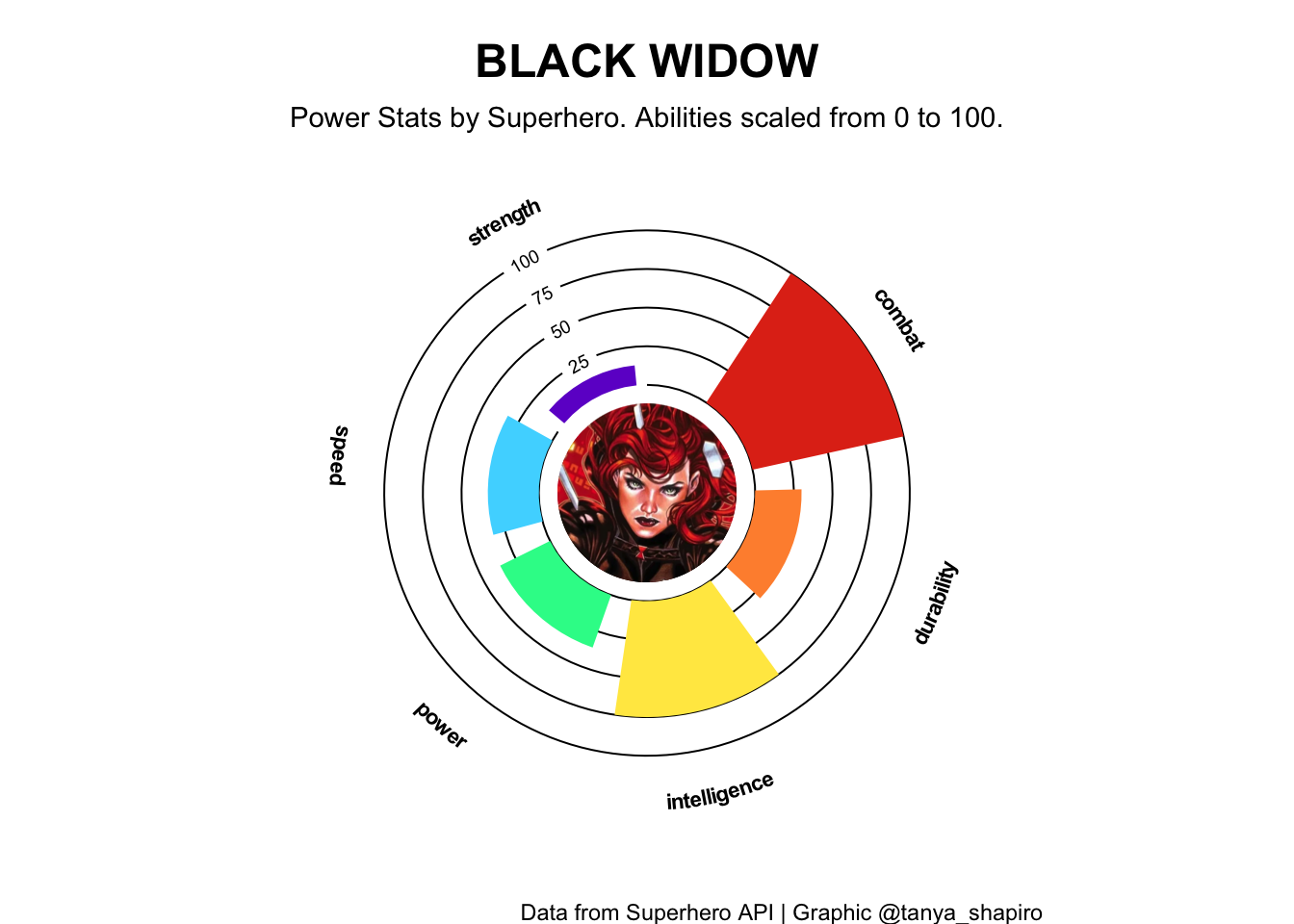How to elevate a radar plot using R `ggplot2` and related extensions.
R
data visualization
ggplot2
Published

August 24, 2022

# Overview

After experimenting with a few different radar plot libraries in R, I wanted to see if I could create a custom radar plot with `ggplot2`. In this tutorial, we’ll cover how to create your own custom `ggplot2` radar graph. Before we begin, here’s a list of the packages we’ll be using:

• tidyverse
• geomtextpath
• ggimage

# Read & Reshape The Data

Let’s kick off with bringing in the data. For our tutorial, we’ll be working with a Superhero data set I collected from an earlier project.

The data set includes information about power abilities per character (intelligence, combat, strength, etc). Before plotting our data, we need to make sure it’s in the right shape- `dplyr` to the rescue! For this exercise, we’ll need it in a long format - each record should represent a super power per character.

When we import `tidyverse`, we automatically import both `dplyr` and `ggplot2`.

``````library(tidyverse)
library(knitr)
library(kableExtra)

#import data

#avengers
avengers<-c(106, 107, 149, 226, 313, 332, 346, 414, 659)

#avengers dataset
df_avengers<-df%>%
filter(id %in% avengers)%>%
select(name, full_name, intelligence, combat, strength, power, speed, durability)%>%
pivot_longer(intelligence:durability, names_to = "ability", values_to = "stat")

#preview data
kable_styling(latex_options = "HOLD_position")``````
name full_name ability stat
Black Panther T'Challa intelligence 88
Black Panther T'Challa combat 100
Black Panther T'Challa strength 16
Black Panther T'Challa power 41
Black Panther T'Challa speed 30
Black Panther T'Challa durability 60

Our data set contains all the Avengers characters. For our tutorial, we’ll pick on one of my favorites to focus on- Black Widow.

To create a basic radar plot, we’ll use `geom_col` and build on this with `coord_polar` - curving our bar on a polar coordinate grid.

``````black_widow <- df_avengers%>%filter(name=="Black Widow")

ggplot(data=black_widow, aes(x=ability,y=stat))+
geom_col()+
coord_polar()``````# Starting from Scratch

To make this truly custom, we’ll need to work with a blank canvas, using ggplot’s `theme_void`. From there, we’ll add back the things we need - namely grid lines and axis labels.

We can add our y grid lines them back in with ggplot’s `geom_segment`.

To create our x axis labels, we will use new ggplot extension package, geomtextpath. This library helps us create curved labels around our radar plot with `geom_textpath`.

``````library(geomtextpath)

#custom dataframe for line segments
segments<- data.frame(
x1=rep(0,5),
x2=rep(5.5,5),
y1=c(0,25,50,75,100),
y2=c(0,25,50,75,100)
)

plot<-ggplot(black_widow, aes(x=ability, y=stat, fill=ability))+
#circular coordinates
coord_polar()+
#blank canvas
theme_void()+
#new x labels
geom_textpath(inherit.aes=FALSE,
mapping=aes(x=ability, label=ability, y=130),
fontface="bold", upright=TRUE, text_only=TRUE, size=3)+
#new grid lines - leave space to add in our y axis labels later
geom_segment(inherit.aes=FALSE,
data = segments,
mapping=aes(x=x1,xend=x2,y=y1,yend=y2), linewidth=0.35)

plot``````## Keep Building

Now that we have our new outline, we’ll overlay our Black Widow data and continue to customize.

Add in the data with ggplot `geom_col`. We’ve already set the aes mapping in the first step, but we’ll use the `width` argument to adjust the column size and suppress the legend by setting `show.legend` to `FALSE.`

To create the donut hole in the center, we’ll use `scale_y_continuous` to adjust the lower limit of our y scale. We’ll also add some padding at the top by creating an upper bound limit.

Finally, we’ll add back in our y axis labels using `geomtextpath::geom_textsegment`.

``````labels<-data.frame(
y = c(25,50,75,100),
x = rep(0.25,4)
)

plot<-plot+
#overlay dataset
geom_col(width=.8, show.legend = FALSE)+
#create donut hole and add some room at the top with limits (our labels are at 130)
scale_y_continuous(limits=c(-70,135))+
geom_textsegment(inherit.aes=FALSE,
data=labels,
mapping=aes(x=5.5, xend=6.5, y= y, yend=y, label=y),
linewidth=0.35, size=2.5)

plot``````## Finishing Touches

Time for finishing touches! We’ll work with one of my favorite R packages, ggimage. Using `geom_image`, we can add png or jpeg images directly in our plot. Let’s go ahead and fill our donut hole with a portrait of Black Widow. I have one ready to use from my GitHub!

We’ll spruce up our bar graph color palette with `scale_fill_manual`. I love playing around with online palette generators like Coolors. You’re welcome to change them with your own hex colors!

Finally, we’ll add our plot titles and labels with ggplot `labs` and format their look and feel with our own `theme`. Put it all together, and it should look something like this:

``````library(ggimage)

image<-'https://raw.githubusercontent.com/tashapiro/superhero-comics/main/images/character-icons/black_widow.png'

plot+
geom_image(mapping=aes(y=-70,x=1), image=image, size=0.225)+
#customize bar colors
scale_fill_manual(values=c("#E1341A","#FF903B","#ffe850","#27f897",
"#4bd8ff" ,"#6F02CE"))+
labs(title="BLACK WIDOW",
subtitle="Power Stats by Superhero. Abilities scaled from 0 to 100.",
caption = "Data from Superhero API | Graphic @tanya_shapiro")+
#make some tweaks to plot theme
theme(
plot.title=element_text(face="bold", hjust=0.5, size=18, vjust=-2),
plot.subtitle=element_text(hjust=0.5, vjust=-5),
)``````## Next Level Stuff

Below is the code for the final Avengers Radar plot I created. Uses all of our ggplot2 tricks and applies them to select characters with ggplot’s `facet_wrap`!

You can check out more Superhero radar plots on my GitHub. If you have any questions, feel free to reach out to me on Twitter @tanya_shapiro. Thank you!

Code
``````#add image links to dataset
df_avengers<-df_avengers%>%
mutate(image = paste0('https://raw.githubusercontent.com/tashapiro/superhero-comics/main/images/character-icons/',tolower(str_replace(name, " ","_")),".png"))

#set color aesthetics as variables
#palettes
pal_font<-"white"
pal_bg <-"#131314"
pal_line <-"#D0D0D0"
pal<-c("#E1341A","#FF903B","#ffe850","#27f897","#4bd8ff","#6F02CE")

ggplot(df_avengers, aes(x=ability, y=stat, fill=ability))+
#create y axis text
geom_textpath(inherit.aes=FALSE,
mapping=aes(x=ability, label=ability, y=130),
fontface="bold", upright=TRUE, text_only=TRUE, linewidth=3, color=pal_font
)+
#image with cahracter icon in the center
geom_image(mapping=aes(y=-70,x=1,image=image), size=0.225)+
#text for actual name below superhero name
geom_text(inherit.aes=FALSE,
mapping=aes(label=full_name, x=1, y=-70),
vjust=-13.6, color="white", size=3.25)+
#create curved coordinate system, curvedpolar accomodates text
coord_curvedpolar()+
#create linesegments to represent panel gridlines
geom_segment(inherit.aes=FALSE,
data = segments,
mapping=aes(x=x1,xend=x2,y=y1,yend=y2),
linewidth=0.45, color=pal_line)+
#bars
geom_col(show.legend = FALSE, width=.8)+
#text for panel gridlines
geom_textsegment(inherit.aes=FALSE,
data=labels,
mapping=aes(x=5.5, xend=6.5, y= y, yend=y, label=y),
color = pal_line, textcolour= pal_font, linewidth=0.45, size=2.5)+
#adjist scales for fill (custom palette) & create y scale limits (create blank circle at center to store image)
scale_fill_manual(values=pal)+
scale_y_continuous(limits=c(-70,135))+
#iterate per character
facet_wrap(~toupper(name))+
labs(title="THE AVENGERS",
subtitle="Power Stats by Superhero. Abilities scaled from 0 to 100.",
caption="Data from Superhero API | Graphic @tanya_shapiro")+
theme_minimal()+
theme(text=element_text(color=pal_font),
plot.background = element_rect(fill=pal_bg),
plot.title=element_text(face="bold", hjust=0.5, size=18, margin=margin(t=5)),
plot.subtitle=element_text(hjust=0.5, margin=margin(t=5, b=20)),
plot.caption = element_text(margin=margin(t=15)),
axis.title=element_blank(),
panel.grid = element_blank(),
plot.margin=margin(t=10,b=5,l=10,r=10),
axis.text=element_blank(),
axis.ticks = element_blank(),
strip.text=element_text(face="bold", color=pal_font, size=12, vjust=-0.5))``````